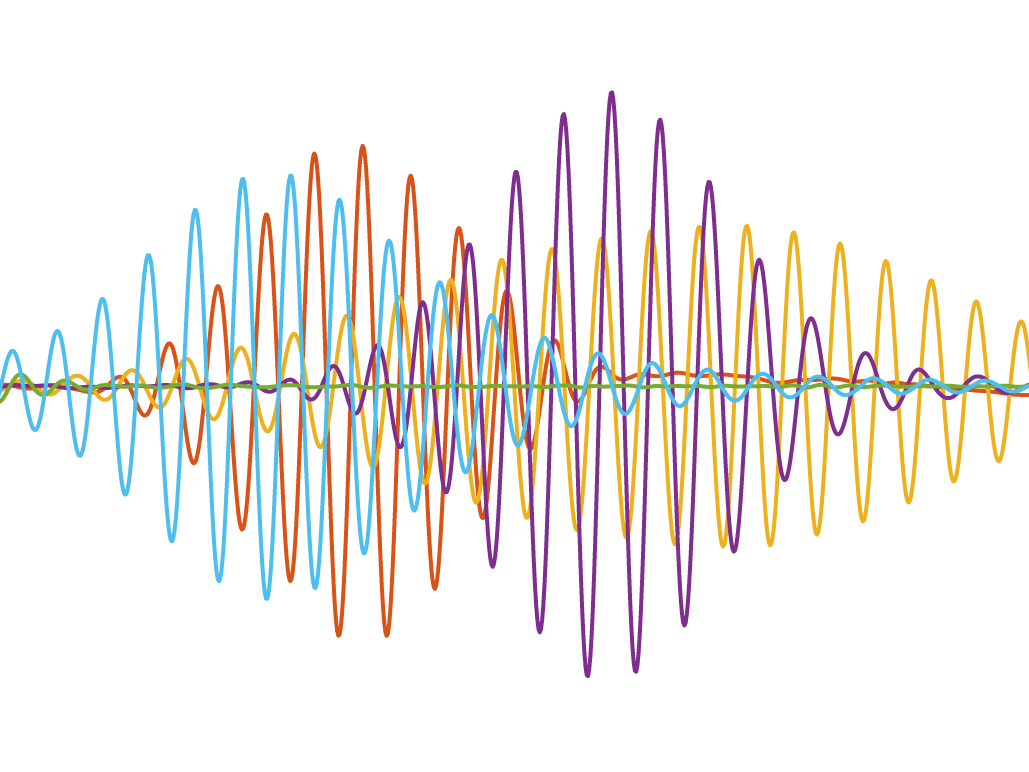# 2D-FFT Analysis in MATLAB

2D-FFT can be a really powerful tool in identifying the propagation of different Lamb wave modes in a signal.Example of a multi-modal signal

2D-FFT can be a really powerful tool in identifying the propagation of different Lamb wave modes in a signal. The process was pioneered by Alleyne and Cawley (1990), and has since been used for a wide range of applications. In this post I will explain how 2D-FFT can be implemented in MATLAB, and how the results can be compared against dispersion curves.

Firstly, the data. This needs to be in the form of equally spaced time-domain samples. In the provided example data here, a COMSOL model has been used to simulate wave propagation through a 2.5 mm thick aluminium plate. Excitation is a 5-cycle Hamming windowed pulse centred at 1 MHz. Wedge transducers have been used to target the A1 mode. Ninety (90) y-axis displacement signals are captured, with a spacing of 0.8 mm. The sampling rate of 8.4×107 is determined by the mesh size of the model, ensuring an adequate resolution.

In the code below, the file is read and converted to an array, where the header and time column are omitted, leaving only the 90 columns of data. An option to filter the data is included, using a bandpass filter.

``````myfile = uigetfile('*.csv','MultiSelect','on');

A1 = table2array(M1);
A1(1,:) = [];

Fs = 1/((A1(2,1))-(A1(1,1)));   %sample rate
St = 1/Fs;     % sampling period
A1 = A1(:,2:end); % remove time column
%filterbw = [0.8e6 2e6]; %filter bandwidth
%A1 = bandpass(A1, filterbw, Fs,'ImpulseResponse','iir'); % filter``````

``````A1 = padarray(A1,[100000 2000], 0,'post'); % zero pad
``````

Now the number of samples, spacing between measurement points, and the sampling period are used to calculate the wavenumber and frequency increments for plotting.

``````Nx = size(A1,2); % Number of samples collected along first dimension
Nt = size(A1,1); % Number of samples collected along second dimension
dx = 0.0008;  % Distance increment (i.e., Spacing between each column)
dt = St; % Time increment (i.e., Spacing between each row)
Nyq_k = 1/dx; % Nyquist of data in first dimension
Nyq_f = 1/dt; % Nyquist of data in second dimension
dk = 1/(Nx*dx);   % Wavenumber increment
df = 1/(Nt*dt);   % Frequency increment
k = -Nyq_k/2:dk:Nyq_k/2-dk; % wavenumber (m)
kr = k*(2*pi)/1000; % wavenumber (rad/mm)
f =-Nyq_f/2:df:Nyq_f/2-df; % frequency (Hz)
krhalf = kr(:,((size(kr,2))+1)/2:end); % take positive quadrant of kr
fhalf = f(:,((size(f,2))+1)/2:end); % take positive quadrant of f``````

Next `fft2` is used to process the data, and the result is reorientated. An absolute value of the result is calculated, and we throw away the data that isn’t positive in both dimensions. The data is also normalised to values between 0 and 1, which helps in applying a `colormap` to the data.

``````fft2result = fftshift(fft2(A1))*dx*dt; %fft2
fft2resultB =fft2result.'; %transpose
fft2resultB = flip(fft2resultB,2); %flip

z = abs(fft2resultB); %absolute value

fft2data = z((size(z,1)+1)/2:end,(size(z,2)+1)/2:end); % take positive quadrant of z

znorm = rescale(fft2data,0,1); % normalise 0-1``````

Now we can plot the result. I am using the `viridis_white` colormap, which helps with readability, as well being more suitable for publication than large blocks of colour.

``````imagesc(fhalf/1e6,krhalf,znorm);
set(gca,'YDir','normal')
colorbar;
colormap(flipud(colormap('viridis_white')))

xlim([0 2])
ylim([0 3])

xlabel('Frequency (MHz)')
hold on
%grid on
box on
ax = gca;
ax.LineWidth = 2;

x0=0;
y0=0;
width=1200;
height=1200;
set(gcf,'position',[x0,y0,width,height])

set(gcf, 'Color', 'w');
``````

The excellent `export_fig` package can be used to export the plots as images.

``export_fig 2DFFT.png -nocrop``

To determine which modes are represented in the plot we can overlay dispersion curves for comparison. I have generated dispersion curves for aluminium using the dispersion calculator and exported them as .mat files. Download them here. Import these tables (A_Lamb and S_Lamb) into your workspace and use the following code to plot them.

``````multiplier = 2.5;

The multiplier is used to correct for the data being exported in terms of frequency-thickness product, while our plate is 2.5 mm thick.

Now the presence of the A1 mode is confirmed, along with the S0 mode.

Thanks to Sarah Johannesmann for her help with the code!